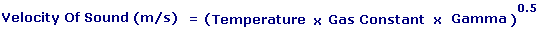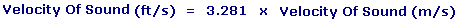Velocity of Sound Enter value and click on calculate. Result will be displayed.Enter your values: Temperature: Kelvin Gamma: Gas Constant: J/kg*K Results: Velocity of Sound: m/s Velocity of Sound: ft/s

 Web www.calculatoredge.com
 Sound is a vibration that travels through an elastic medium as a wave. The Velocity of Sound describes how much distance such a wave travels in a given amount

In Dry Air with a temperature of 21 °C (70 °F) the Speed of Sound is 344 m/s (1230 km/h, or 770 mph, or 1130 ft/s). Although it is commonly used to refer specifically to air, the speed of sound can be measured in virtually any substance. The Velocity of Sound in liquids and non-porous solids is much higher than that in air.

In the Earth's atmosphere the most important factor affecting the Velocity of Sound is the temperatureLINKSDISCLAIMERCONTACT US